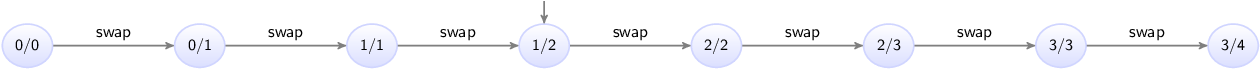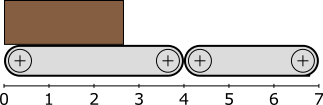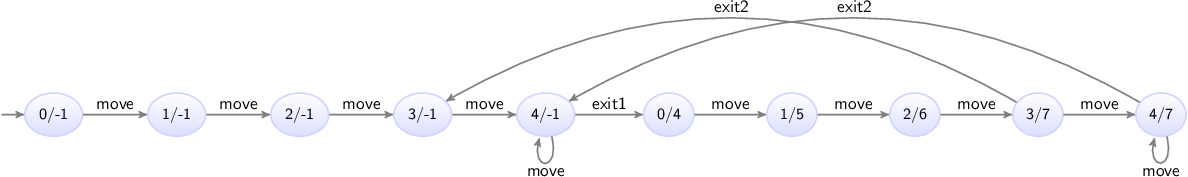## Old and new values in assignments

This lesson explains old and new values of variables in assignments, multiple assignments, and the order of assignments.

### Old and new values

Consider the following CIF specification:

``````automaton counter:
event increment;

disc int count = 0;

location:
initial;
edge increment do count := count + 1;
end``````

The `counter` automaton represents a counter that starts counting at zero, and can be incremented in steps of one.

In assignments, the part to the left of the `:=` is called the left hand side of the assignment, or the addressable. The addressable is the variable that is assigned, and gets the new value. In the example above, variable `count` is assigned a new value.

The part to the right of the `:=` is called the right hand side of the assignment, or the (new) value. In the example above, the new value is computed by taking the current or old value of variable `count` and incrementing it by one.

In general, for variables used to compute the new value, always the old value of those variables are used. The new values for variables after a transition, are always computed from the old values of variables from before that transition.

### Multiple assignments

It is allowed to update multiple variables on a single edge, leading to multiple variables getting a new value as part of a single transition. For instance, consider the following CIF specification:

``````automaton swapper:
event swap;
disc int x = 0, y = 0;

location:
initial;
edge swap do x := y, y := x + 1;
end``````

The `swapper` automaton declares two variables, `x` and `y`. It keeps swapping the values of both variables, each time increasing the value of `y` by one.

Initially, both variables have value zero. During the first `swap`, variable `x` gets the value of variable `y`. Since the old values of the variables are used to compute the new values, variable `x` remains zero. Variable `y` gets the old value of variable `x`, which is also zero, incremented by one. The result of the first swap is that `x` remains zero and `y` becomes one.

During the second swap, `x` gets the value of variable `y`, which is then one. Variable `y` gets the value of variable `x`, which was still zero before the second swap, incremented by one. Both variables are thus one after the second swap.

During the third swap, `x` gets the value of variable `y` from after the second swap, and thus remains one. Variable `y` becomes two.

The state space of this somewhat artificial example is as follows:The states are labeled with the values of variables `x` and `y`.

### Assignment order

It is important to note that since the new values of the variables are computed from the old values of the variables, assignments are completely independent of each other. In the example above, variable `x` is assigned a new value in the first assignment, and variable `x` is also used to compute the new value of variable `y`. However, the old value of variable `x` is used to compute the new value of variable `y`. Therefore, the assignment to `x`, which indicates how `x` should be given a new value, has no effect on the new value off `y`, as the old value of `x` is used for that, regardless of whether `x` is assigned a new value.

Since assignments are independent of each other, the order of the assignments of the edge does not matter. Consider the following alternative edge:

``edge swap do y := x + 1, x := y;``

The assignments to `x` and `y` have been reordered. The behavior of the specification does not change as a result of this reordering.

### Multi-assignments

CIF supports both multiple assignments as well as multi-assignments. To see the difference, consider the following examples:

``````edge ... do x := y, y := x + 1;     // Multiple (two) assignments.
edge ... do (x, y) := (y, x + 1);   // Single multi-assignment.``````

The first edge has multiple assignments, namely one assignment to variable `x` and one assignment to variable `y`. The second edge has one assignment, that gives new values to variables `x` and `y`. Both are identical, in that they have the same affect: variable `x` is given the old value of variable `y` and variable `y` is given the old value of variable `x` incremented by one. Generally, using multiple assignments is preferred over using multi-assignments, as the former is easier to read. However, in certain cases, such as for tuple unpacking, only the latter variant can be used.

### Event synchronization and assignment order

Consider a system with two conveyors. Products enter on the first conveyor, and move towards the second conveyor. Once they leave the first conveyor, they move onto the second one. Once they exit from the second conveyor, they leave the system. The positions of the left sides of the boxes are in range zero to seven, as indicated in the following figure:This system can be modeled using the following CIF specification:

``````event move;

automaton conveyor1:
monitor move;
event exit1;
disc int pos = 0;

location:
initial;
edge move  when pos < 4 do pos := pos + 1;
edge exit1 when pos = 4 do pos := 0;
end

automaton conveyor2:
monitor move;
event exit2;
disc int pos = -1;

location:
initial;
edge conveyor1.exit1 when pos = -1             do pos := conveyor1.pos;
edge move            when pos >= 0 and pos < 7 do pos := pos + 1;
edge exit2           when pos = 7              do pos := -1;
end``````

Each conveyor is modeled using an automaton. Both conveyors use a `pos` variable to represent the position of the left side of the box. The first conveyor gets a new box as soon as one leaves. The second one has to wait for a box from the first, and can thus be without a box. This is represented by value `-1` for the `pos` variable from automaton `conveyor2`. The `-1` value is not a actual position, but a special value indicating that no box is present on the conveyor.

Boxes on the first conveyor can move towards the second conveyor (event `move`), until they reach position 4. They then leave the first conveyor (event `exit1`), and a new box immediately enters the first conveyor (variable `pos` is reset to zero).

Boxes enter the second conveyor when they leave the first conveyor (event `exit1` from `conveyor1`). The position of the box is then transferred from the first conveyor to the second. The box keeps moving (event `move`) on the second conveyor until it reaches position 7. At position 7 it leaves (event `exit2`) the second conveyor, and the system.

Both automata synchronize over the `move` event, meaning that the boxes on both conveyors move at the same time. Both automata monitor that event to ensure it is never blocked if only the other conveyor can actually move.

Both automata synchronize over the `exit1` event. The first conveyor resets is own position (variable `pos`) to zero. The second conveyor sets its own position (variable `pos`) to the position of the first conveyor. Since old values of variables are used to compute the new values, the new value of variable `pos` in `conveyor2` is given the old value of variable `pos` from `conveyor1`. This is not influenced by the assignment to variable `pos` of `conveyor1` to zero, as assignments are independent, and the order of assignments does not matter, just as for multiple assignments on a single edge.

The state space of this specification is as follows:The states are labeled with the values of the `pos` variables of the automata for the first and second conveyors.

The important part of the state space is the transition from state `4/-1`, where the box of the first conveyor is at the end and the second conveyor has no box, to state `0/4`, where the first conveyor has received a new box at position zero, and the second conveyor has taken over the box (and the administration of its position) from the first conveyor.